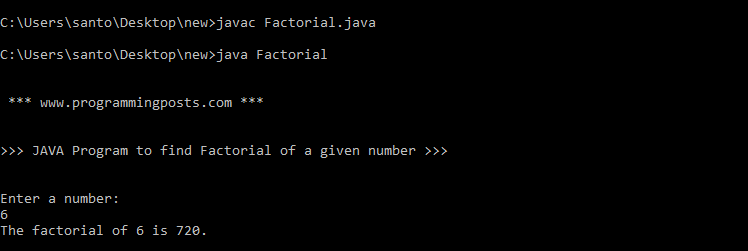# Java program to find factorial of a given number

The Factorial of a non-negative number n is the product of all positive integers less than or equal to n and is represented by n!.

n! = 1 * 2 * 3 * 4 * 5 * ………………………..  * (n-1) * n.

for example

5! can be calculated as 1 * 2 * 3 * 4 * 5 =120.

The value of 0! is 1 according to Empty convention.

### Algorithm:

Step 1: START.

Step 2: Read Number n.

Step 3: Initialize two variables, say i = 1 and fact = 1.

Step 4: fact = fact * i.

Step 5: i = i+1.

Step 6: Repeat step 4 and 5 until i=n.

Step 7:  Print fact.

Step 8: STOP.

### Source code:

```import java.util.Scanner;
class Factorial
{
public static void main(String args[])
{
System.out.println("\n \n *** www.programmingposts.com *** \n \n");
System.out.println(">>> Addition of two numbers without using Third Variable <<< \n\n ");
Scanner scanner = new Scanner(System.in);
System.out.println("Enter a number:");
int num = scanner.nextInt();
int i = 1;
int fact = 1;
for( i = 1; i <= num; i++)
{
fact = fact * i;
}
System.out.println("The factorial of " + num + " is " +fact +"." );
}
}```

### Explanation:

Let the number whose  factorial is to be found be stored in num.

A new variable “fact” is declared as int data type and is intialized to 1 i.e., fact =1.

Now, we need to multiply the variable “fact” with all the natural numbers 1 to num.

For this purpose, we use a for loop with a counter i that ranges from 1 to num. Within the loop, the existing value of fact will be multiplied with the loop counter.

for (int i = 1; i <= num; i++) {
fact = fact * i;
}
To understand how the above loop works ,Let us take a small number num = 3 . Before entering the loop, fact would be initialized to one.

The loop will execute thrice with the value of i = 1, 2 and 3. When the value of i becomes 4, the loop condition fails. When the value of i is 1, the existing fact would be multiplied with 1 which again gives one. In the second iteration, fact will be multiplied with 2 and in the third iteration with 3. These calculations are shown below:

fact = 1
i = 1           fact = fact * i = 1 * 1 = 1
i = 2           fact = fact * i = 1 * 2 = 2
i = 3           fact = fact * i = 2 * 3 = 6

if we enter the number as 0, it doesn’t get  into the loop for iteration and it directly prints the fact value which is initialized to 1.

### Output:

C:\Users\santo\Desktop\new>javac Factorial.java

C:\Users\santo\Desktop\new>java Factorial

*** www.programmingposts.com ***

>>> JAVA Program to find Factorial of a given number <<<

Enter a number:
6
The factorial of 6 is 720.

### Screenshot of Output:screenshot of output